# Intel景深攝影機Python程式詳解2-於NVIDIA Jetson Nano執行RealSense D435 範例

 作者 張嘉鈞 難度 普通 材料表 Intel RealSense D435 X1NVIDIA Jetson Nano X1

### 對齊RGB跟Depth畫面並去除背景 ( align-depth2color.py )

``````# 導入函式庫
import pyrealsense2 as rs
import numpy as np
import cv2
# 建立一個context物件存放所有 RealSense 的處理函示
pipeline = rs.pipeline()
# 配置串流物件
config = rs.config()
``````

``````# 可以透過下列程式取得設備資訊
# 透過 resolve 確認第一個可用的設備
pipeline_wrapper = rs.pipeline_wrapper(pipeline)
pipeline_profile = config.resolve(pipeline_wrapper)
# 取得該設備資訊
device = pipeline_profile.get_device()
device_product_line = str(device.get_info(rs.camera_info.product_line))
# 建立「深度」影像串流
config.enable_stream(rs.stream.depth, 640, 480, rs.format.z16, 30) # uint 16
# 建立「彩色」影像串流
# 由於 L500 系列的彩色攝影機解析度維 960 x 540 所以才需要取得設備資訊
if device_product_line == 'L500':
config.enable_stream(rs.stream.color, 960, 540, rs.format.bgr8, 30)
else:
config.enable_stream(rs.stream.color, 640, 480, rs.format.bgr8, 30)
# 開啟影像串流
profile = pipeline.start(config)
``````

``````# 取得深度感測器物件
depth_sensor = profile.get_device().first_depth_sensor()
# 取得深度感測器的 深度資訊與實際公尺之間的 映射尺度
# get_depth_scale -> Retrieves mapping between the units of the depth image and meters
depth_scale = depth_sensor.get_depth_scale()
print("Depth Scale is: " , depth_scale)
# 宣告多少公尺以內的資訊要顯示
clipping_distance_in_meters = 1 #1 meter
# 將 公尺資訊 轉換成 RealSense 的 深度單位
clipping_distance = clipping_distance_in_meters / depth_scale
``````

``````# 建立 對齊 物件 ( 以 color 為基準 )
align_to = rs.stream.color
# rs.align 讓 深度圖像 跟 其他圖像 對齊
align = rs.align(align_to)
# 使用 process 來實現剛剛宣告的 align 對齊功能
# aligned_frames = align.process(frames)
``````

``````# 串流迴圈
try:
while True:
# 等待最新的影像，wait_for_frames返回的是一個合成的影像
frames = pipeline.wait_for_frames()
# frames.get_depth_frame() is a 640x360 depth image
# 使用 process 來實現剛剛宣告的 align 對齊功能
aligned_frames = align.process(frames)
``````

``````        # 取得對齊後的影像
depth_frame = frames.get_depth_frame()
aligned_depth_frame = aligned_frames.get_depth_frame() # aligned_depth_frame is a 640x480 depth image
color_frame = aligned_frames.get_color_frame()
# 驗證是否都有影像
if not aligned_depth_frame or not color_frame:
continue
# 轉換成 numpy
depth_image = np.asanyarray(aligned_depth_frame.get_data())
color_image = np.asanyarray(color_frame.get_data())
# 顯示解析度
# print('Org (L500) : ', np.shape(np.asanyarray(depth_frame.get_data())), end='\t')
# print('After align : ', np.shape(np.asanyarray(aligned_depth_frame.get_data())))
``````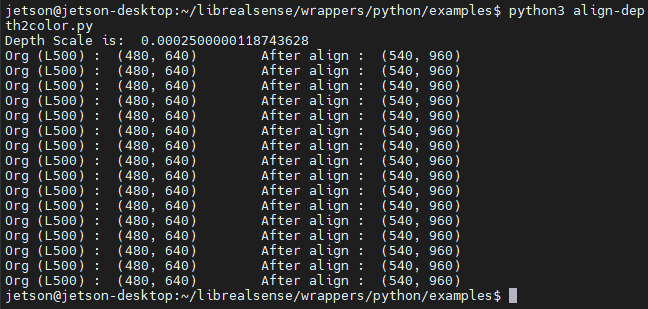``````        # 刪除背景 : 將背景顏色調整成 灰色
grey_color = 153
# 深度影像只有 1 個 channel，透過 dstack 建立 灰階圖 ( 這邊RGB的數值就是 深度訊息 )
depth_image_3d = np.dstack((depth_image, depth_image, depth_image)) #depth image is 1 channel, color is 3 channels
# 當 深度大於 1 公尺 或是 偵測不出來 就會 以 grey_color 取代
bg_removed = np.where((depth_image_3d > clipping_distance) | (depth_image_3d <= 0), grey_color, color_image)
``````

``````        # 將深度影像轉換成 彩色影像
depth_colormap = cv2.applyColorMap(cv2.convertScaleAbs(depth_image, alpha=0.03), cv2.COLORMAP_JET)
# 合併圖像 左為去背圖，右為深度圖
images = np.hstack((bg_removed, depth_colormap))
# cv2.namedWindow('Align Example', cv2.WINDOW_NORMAL)
cv2.imshow('Align Example', images)
key = cv2.waitKey(1)
# Press esc or 'q' to close the image window
if key & 0xFF == ord('q') or key == 27:
cv2.destroyAllWindows()
break
finally:
pipeline.stop()
``````

``````import numpy as np
a = np.asanyarray( [[8000, 2000],
[0, 125]])
b = np.dstack((a,a,a))
print(a.shape, b.shape)
print('\n')
print(a)
print('\n')
print(b)
``````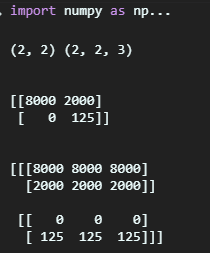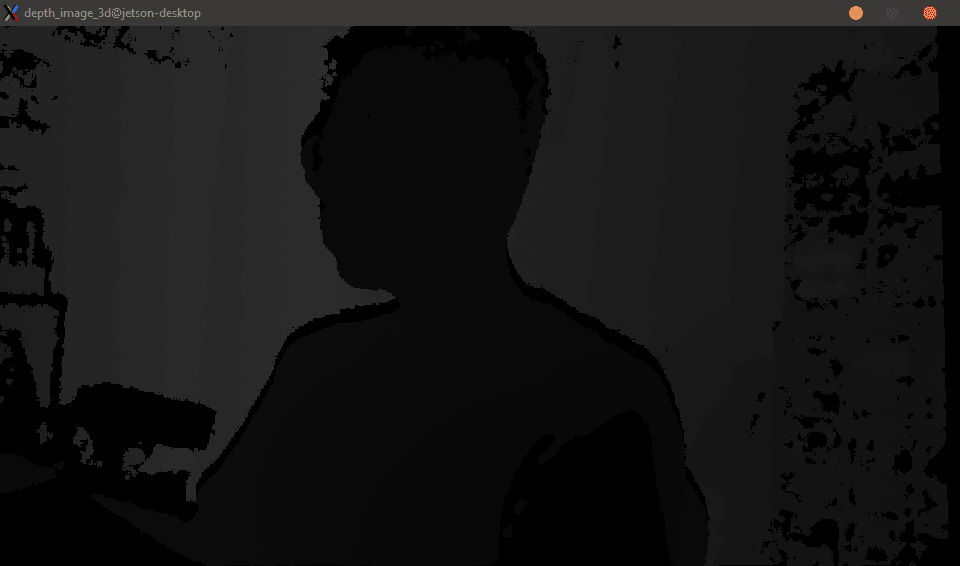### 解決掉幀問題 ( frame_queue_example.py )

``````# First import the library
import pyrealsense2 as rs
import time
# 每20個frame會暫停0.25秒 製造短暫的延遲
def slow_processing(frame):
n = frame.get_frame_number()
if n % 20 == 0:
time.sleep(1/4)
print(n)
# 製造更延遲的狀況
def slower_processing(frame):
n = frame.get_frame_number()
if n % 20 == 0:
time.sleep(1)
print(n)
``````

``````try:
# Create a pipeline
pipeline = rs.pipeline()
# Create a config and configure the pipeline to stream
#  different resolutions of color and depth streams
config = rs.config()
config.enable_stream(rs.stream.depth, 640, 480, rs.format.z16, 30)
``````

``````    print("Slow callback")
# 開始影像串流
pipeline.start(config)
# 讓 slow_processing 運行5秒就好
start = time.time()
while time.time() - start < 5:
# 取得最新的影像物件
frames = pipeline.wait_for_frames()
# 運行slow_processing
slow_processing(frames)
# 關閉RealSense物件
pipeline.stop()
``````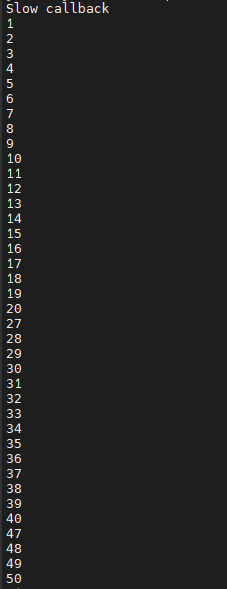``````    # 加上 queue
print("Slow callback + queue")
# frame_queue(n) n 是可以保留多少幀，這邊保留了50幀的空間
queue = rs.frame_queue(50)
# 開啟串流
pipeline.start(config, queue)
# 執行五秒
start = time.time()
while time.time() - start < 5:
frames = queue.wait_for_frame()
# 一樣使用 slow_processing
slow_processing(frames)
``````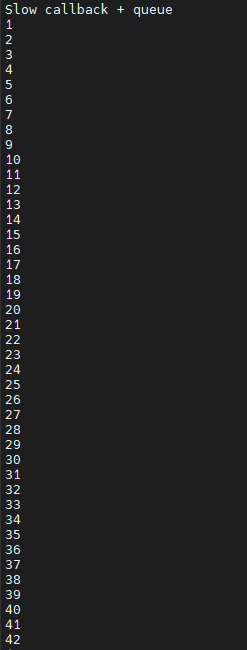``````    print("Slower callback + queue")
queue = rs.frame_queue(50)
pipeline.start(config, queue)
start = time.time()
while time.time() - start < 5:
frames = queue.wait_for_frame()
slower_processing(frames)
pipeline.stop()
``````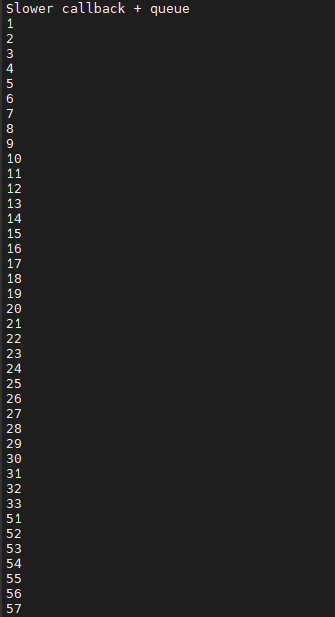``````    print("Slower callback + keeping queue")
queue = rs.frame_queue(50, keep_frames=True)
pipeline.start(config, queue)
start = time.time()
while time.time() - start < 5:
frames = queue.wait_for_frame()
slower_processing(frames)
pipeline.stop()
``````

### 錄製深度影像 (.bag)

``````# 建立一個context物件存放所有 RealSense 的處理函示
pipeline = rs.pipeline()
# 配置串流物件
config = rs.config()
# 建立「深度」影像串流
config.enable_stream(rs.stream.depth, 640, 480, rs.format.z16, 30)
# 將影像數據輸出到 test.bag 當中
config.enable_record_to_file('test.bag')
``````

### 讀取預錄好的深度影像方式

``````import pyrealsense2 as rs
import numpy as np
import cv2
import argparse
import os.path
try:
pipeline = rs.pipeline()
config = rs.config()
# 告訴 config 資訊從檔案讀取
rs.config.enable_device_from_file(config, 'test.bag')
# 一樣要建立深度影像串流
config.enable_stream(rs.stream.depth, rs.format.z16, 30)
# 開啟串流
pipeline.start(config)
# 宣告著色器 預設是 jet colormap
colorizer = rs.colorizer();
``````

``````    while True:
frames = pipeline.wait_for_frames()
depth_frame = frames.get_depth_frame()
# 將深度影像著色成 jet colormap
depth_color_frame = colorizer.colorize(depth_frame)
# 轉換成 numpy array 讓 opencv 顯示
depth_color_image = np.asanyarray(depth_color_frame.get_data())
# Opencv 顯示以及按下 q 離開
cv2.imshow("Depth Stream", depth_color_image)
key = cv2.waitKey(1)
if key == 27:
cv2.destroyAllWindows()
break
finally:
pass
``````

### 結語

CAVEDU Education is devoted into robotics education and maker movement since 2008, and is intensively active in teaching fundamental knowledge and skills. We had published many books for readers in all ages, topics including Deep Learning, edge computing, App Inventor, IoT and robotics. Please check CAVEDU's website for more information: http://www.cavedu.com, http://www.appinventor.tw